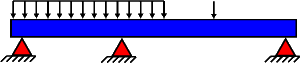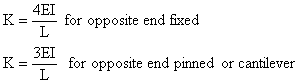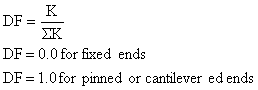﻿ Moment Distribution

# Moment DistributionMoment distribution is an analysis method which can be used to determine the bending moment distribution in an indeterminate beam.

## Analysis Method:

1.  Calculate stiffness factors for each member1.  Calculate distribution factors at both ends of each member1.  Determine carryover factors at both ends of each member
COF = 0.5 for opposite end fixed
COF = 0.0 for opposite end pinned or cantilevered
1.  Assume all joints are fixed and calculate fixed-end moments for each member.

2. Balance pinned (to zero) and cantilevered ends and distribute half the moment to the opposite end.

3. Distribute the unbalanced moments at all other joints to each adjacent member based on the distribution factor.

4. Carryover the distributed moments to the opposite ends of the each member using the carryover factors.

5. Iterate steps 6 and 7 until moment imbalance at each joint approaches zero.

6. For unbraced frames, solve equilibrium equations for the restraining force and apply an equal and opposite force to the frame.  Calculate moments due to this force and combine them with those from the braced frame to get the total moments.

## Reference Documents:

1. Fundamentals of Structural Analysis, West, John Wiley & Sons.
2. Introductory Structural Analysis, Wang & Salmon
3. Structural Analysis, Laursen, McGraw-Hill